# Array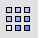Transform

Array >

RectangularHistory enabled

The Array command copies objects spaced in columns, rows, and levels in the x, y, and z directions.

#### Steps

1. Select the objects.
The array directions are the x, y, and z directions of the active construction plane.
2. Type the number of copies in the x direction, and press Enter.
3. Type values of 1 or more for the number of copies.
4. Type the number of copies in the y direction.
5. Type the number of copies in the z direction.
6. Pick the corners of a rectangle that defines the unit cell (x and y spacing) distance.
7. Pick the height of the unit cell, or press Enter to use the width distance.
Or
Specify the distances
for the x spacing, y spacing, and z spacing.
8. Press Enter to accept the array or change the options.

Command-line options

##### Number

Specifies the number of objects in the array.

##### XNumber / YNumber / ZNumber

Changes the number of objects in the x, y, and z directions.

##### Preview

Displays a dynamic preview. You can change the options and the preview will update.

##### Spacing

Specifies the spacing between objects in the array.

##### XSpacing / YSpacing / ZSpacing

Changes the spacing in the x, y, and z directions.

# ArrayLinear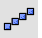Transform

Array >

Linear

The ArrayLinear command copies and spaces objects in a single direction.

#### Steps

1. Select objects to array.
2. Pick the first reference point.
3. Pick the second reference point.
The direction of the array and the distance between the objects is determined by the second pick point.
Command-line options

Number

The number of total objects resulting from the array.

Preview

Displays a dynamic preview. You can change the options and the preview will update.

# ArrayPolar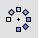Transform

Array >

PolarHistory enabled

The ArrayPolar command copies objects around a central location.

#### Steps

1. Select the objects.
2. Pick the center of the array.
The rotation axis for the polar array is the z direction of the active construction plane at the chosen point.
3. Type the number of items and press Enter.
4. Enter a value of 2 or more.
5. Type the angle to fill.
Copies of the selected objects appear, rotated around the axis defined by the center point.

Command-line options

##### Axis

Defines an axis line for the array to rotate around.

#### Axis steps

1. Pick the start of polar axis.
2. Pick the end of polar axis.
The action is similar to the Rotate3D command.
Press Enter to rotate the array around the construction plane z axis.
##### UseLastAxisUses the previously selected axis direction for the rotation.

##### Preview

Displays a dynamic preview. You can change the options and the preview will update.

##### StepAngle

Enter the angle between objects.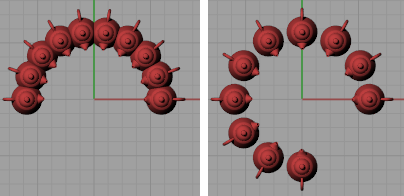StepAngle=20 (left) and StepAngle=30 (right).
##### Rotate

Rotates the objects as they are arrayed.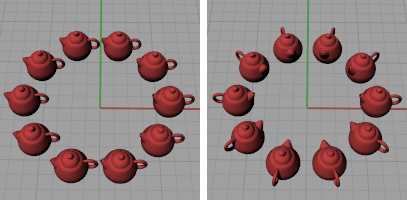Rotate=No (left) and Rotate=Yes (right).
##### ZOffset

Moves each arrayed object in the z direction by the value specified.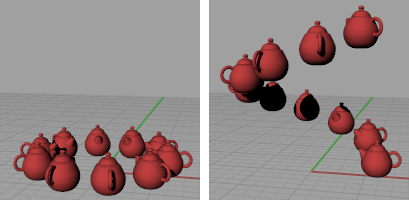ZOffset=0 (left) and ZOffset=1 (right).
• Type a negative value to offset objects in the negative CPlane-Z direction.